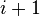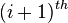# Parity progression ratio

## Definition

The term parity progression ratio (PPR) is typically used in the context of a cohort of females (such as a birth cohort) that has completed its childbearing years. Given such a cohort, and a nonnegative integer$i$, the parity progression ratio from birth order$i$ to birth order$i + 1$ is defined as the ratio of the completed cohort fertility for that cohort for birth order$i+1$, to the completed cohort fertility for that cohort for birth order$i$.

Alternatively, the PPR describes the probability that a person in that cohort who has had$i$ children will proceed to have an$(i+1)^{th}$ child.